# highpass

Highpass-filter signals

## Syntax

``y = highpass(x,wpass)``
``y = highpass(x,fpass,fs)``
``y = highpass(xt,fpass)``
``y = highpass(___,Name,Value)``
``[y,d] = highpass(___)``
``highpass(___)``

## Description

````y = highpass(x,wpass)` filters the input signal `x` using a highpass filter with normalized passband frequency `wpass` in units of π rad/sample. `highpass` uses a minimum-order filter with a stopband attenuation of 60 dB and compensates for the delay introduced by the filter. If `x` is a matrix, the function filters each column independently.```

example

````y = highpass(x,fpass,fs)` specifies that `x` has been sampled at a rate of `fs` hertz. `fpass` is the passband frequency of the filter in hertz.```
````y = highpass(xt,fpass)` highpass-filters the data in timetable `xt` using a filter with a passband frequency of `fpass` hertz. The function independently filters all variables in the timetable and all columns inside each variable.```

example

````y = highpass(___,Name,Value)` specifies additional options for any of the previous syntaxes using name-value pair arguments. You can change the stopband attenuation, the Highpass Filter Steepness, and the type of impulse response of the filter.```

example

````[y,d] = highpass(___)` also returns the `digitalFilter` object `d` used to filter the input.```
````highpass(___)` with no output arguments plots the input signal and overlays the filtered signal.```

## Examples

collapse all

Create a signal sampled at 1 kHz for 1 second. The signal contains two tones, one at 50 Hz and the other at 250 Hz, embedded in Gaussian white noise of variance 1/100. The high-frequency tone has twice the amplitude of the low-frequency tone.

```fs = 1e3; t = 0:1/fs:1; x = [1 2]*sin(2*pi*[50 250]'.*t) + randn(size(t))/10;```

Highpass-filter the signal to remove the low-frequency tone. Specify a passband frequency of 150 Hz. Display the original and filtered signals, and also their spectra.

`highpass(x,150,fs)`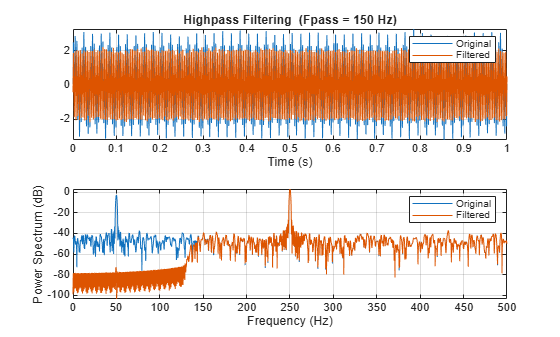Implement a basic digital music synthesizer and use it to play a traditional song. Specify a sample rate of 2 kHz. Plot the spectrogram of the song.

```fs = 2e3; t = 0:1/fs:0.3-1/fs; l = [0 130.81 146.83 164.81 174.61 196.00 220 246.94]; m = [0 261.63 293.66 329.63 349.23 392.00 440 493.88]; h = [0 523.25 587.33 659.25 698.46 783.99 880 987.77]; note = @(f,g) [1 1 1]*sin(2*pi*[l(g) m(g) h(f)]'.*t); mel = [3 2 1 2 3 3 3 0 2 2 2 0 3 5 5 0 3 2 1 2 3 3 3 3 2 2 3 2 1]+1; acc = [3 0 5 0 3 0 3 3 2 0 2 2 3 0 5 5 3 0 5 0 3 3 3 0 2 2 3 0 1]+1; song = []; for kj = 1:length(mel) song = [song note(mel(kj),acc(kj)) zeros(1,0.01*fs)]; end song = song/(max(abs(song))+0.1); % To hear, type sound(song,fs) pspectrum(song,fs,'spectrogram','TimeResolution',0.31, ... 'OverlapPercent',0,'MinThreshold',-60)```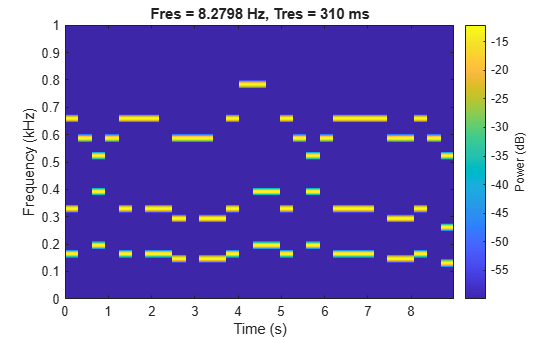Highpass-filter the signal to separate the melody from the accompaniment. Specify a passband frequency of 450 Hz. Plot the original and filtered signals in the time and frequency domains.

```hong = highpass(song,450,fs); % To hear, type sound(hong,fs) highpass(song,450,fs)```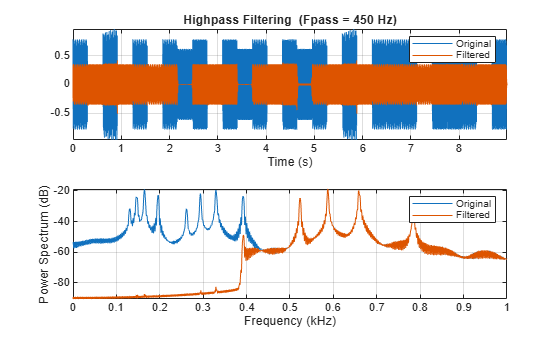Plot the spectrogram of the melody.

```figure pspectrum(hong,fs,'spectrogram','TimeResolution',0.31, ... 'OverlapPercent',0,'MinThreshold',-60)```Filter white noise sampled at 1 kHz using an infinite impulse response highpass filter with a passband frequency of 200 Hz. Use different steepness values. Plot the spectra of the filtered signals.

```fs = 1000; x = randn(20000,1); [y1,d1] = highpass(x,200,fs,'ImpulseResponse','iir','Steepness',0.5); [y2,d2] = highpass(x,200,fs,'ImpulseResponse','iir','Steepness',0.8); [y3,d3] = highpass(x,200,fs,'ImpulseResponse','iir','Steepness',0.95); pspectrum([y1 y2 y3],fs) legend('Steepness = 0.5','Steepness = 0.8','Steepness = 0.95')```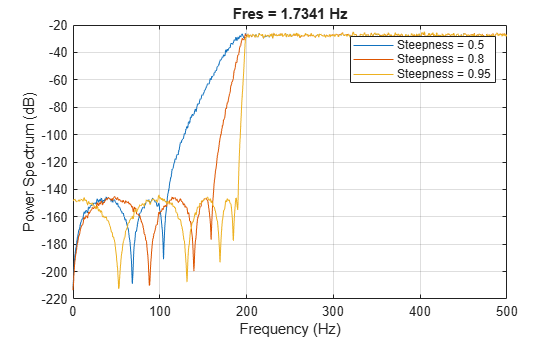Compute and plot the frequency responses of the filters.

```[h1,f] = freqz(d1,1024,fs); [h2,~] = freqz(d2,1024,fs); [h3,~] = freqz(d3,1024,fs); plot(f,mag2db(abs([h1 h2 h3]))) legend('Steepness = 0.5','Steepness = 0.8','Steepness = 0.95') ylim([-130 10])```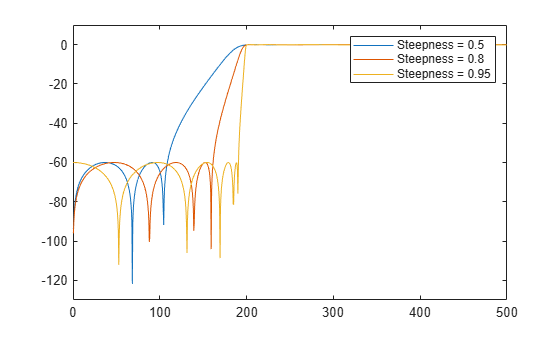## Input Arguments

collapse all

Input signal, specified as a vector or matrix.

Example: `sin(2*pi*(0:127)/16)+randn(1,128)/100` specifies a noisy sinusoid

Example: `[2 1].*sin(2*pi*(0:127)'./[16 64])` specifies a two-channel sinusoid.

Data Types: `single` | `double`
Complex Number Support: Yes

Normalized passband frequency, specified as a scalar in the interval (0, 1).

Passband frequency, specified as a scalar in the interval (0, `fs`/2).

Sample rate, specified as a positive real scalar.

Input timetable. `xt` must contain increasing, finite, and equally spaced row times of type `duration` in seconds.

If a timetable has missing or duplicate time points, you can fix it using the tips in Clean Timetable with Missing, Duplicate, or Nonuniform Times.

Example: `timetable(seconds(0:4)',randn(5,1))` specifies a random variable sampled at 1 Hz for 4 seconds.

### Name-Value Arguments

Specify optional pairs of arguments as `Name1=Value1,...,NameN=ValueN`, where `Name` is the argument name and `Value` is the corresponding value. Name-value arguments must appear after other arguments, but the order of the pairs does not matter.

Before R2021a, use commas to separate each name and value, and enclose `Name` in quotes.

Example: `'ImpulseResponse','iir','StopbandAttenuation',30` filters the input using a minimum-order IIR filter that attenuates frequencies lower than `fpass` by 30 dB.

Type of impulse response of the filter, specified as the comma-separated pair consisting of `'ImpulseResponse'` and `'fir'`, `'iir'`, or `'auto'`.

• `'fir'` — The function designs a minimum-order, linear-phase, finite impulse response (FIR) filter. To compensate for the delay, the function appends to the input signal N/2 zeros, where N is the filter order. The function then filters the signal and removes the first N/2 samples of the output.

In this case, the input signal must be at least twice as long as the filter that meets the specifications.

• `'iir'` — The function designs a minimum-order infinite impulse response (IIR) filter and uses the `filtfilt` function to perform zero-phase filtering and compensate for the filter delay.

If the signal is not at least three times as long as the filter that meets the specifications, the function designs a filter with smaller order and thus smaller steepness.

• `'auto'` — The function designs a minimum-order FIR filter if the input signal is long enough, and a minimum-order IIR filter otherwise. Specifically, the function follows these steps:

• Compute the minimum order that an FIR filter must have to meet the specifications. If the signal is at least twice as long as the required filter order, design and use that filter.

• If the signal is not long enough, compute the minimum order that an IIR filter must have to meet the specifications. If the signal is at least three times as long as the required filter order, design and use that filter.

• If the signal is not long enough, truncate the order to one-third the signal length and design an IIR filter of that order. The reduction in order comes at the expense of transition band steepness.

• Filter the signal and compensate for the delay.

Transition band steepness, specified as the comma-separated pair consisting of `'Steepness'` and a scalar in the interval [0.5, 1). As the steepness increases, the filter response approaches the ideal highpass response, but the resulting filter length and the computational cost of the filtering operation also increase. See Highpass Filter Steepness for more information.

Filter stopband attenuation, specified as the comma-separated pair consisting of `'StopbandAttenuation'` and a positive scalar in dB.

## Output Arguments

collapse all

Filtered signal, returned as a vector, a matrix, or a timetable with the same dimensions as the input.

Highpass filter used in the filtering operation, returned as a `digitalFilter` object.

collapse all

### Highpass Filter Steepness

The `'Steepness'` argument controls the width of a filter's transition region. The lower the steepness, the wider the transition region. The higher the steepness, the narrower the transition region.

To interpret the filter steepness, consider the following definitions:

• The Nyquist frequency, fNyquist, is the highest frequency component of a signal that can be sampled at a given rate without aliasing. fNyquist is 1 (×π rad/sample) when the input signal has no time information, and `fs`/2 hertz when the input signal is a timetable or when you specify a sample rate.

• The stopband frequency of the filter, fstop, is the frequency below which the attenuation is equal to or greater than the value specified using `'StopbandAttenuation'`.

• The transition width of the filter, W, is `fpass`fstop, where `fpass` is the specified passband frequency.

• Most nonideal filters also attenuate the input signal across the passband. The maximum value of this frequency-dependent attenuation is called the passband ripple. Every filter used by `highpass` has a passband ripple of 0.1 dB.When you specify a value, s, for `'Steepness'`, the function computes the transition width as

W = (1 – s) × `fpass`.

• When `'Steepness'` is equal to 0.5, the transition width is 50% of `fpass`.

• As `'Steepness'` approaches 1, the transition width becomes progressively narrower until it reaches a minimum value of 1% of `fpass`.

• The default value of `'Steepness'` is 0.85, which corresponds to a transition width that is 15% of `fpass`.

## Version History

Introduced in R2018a